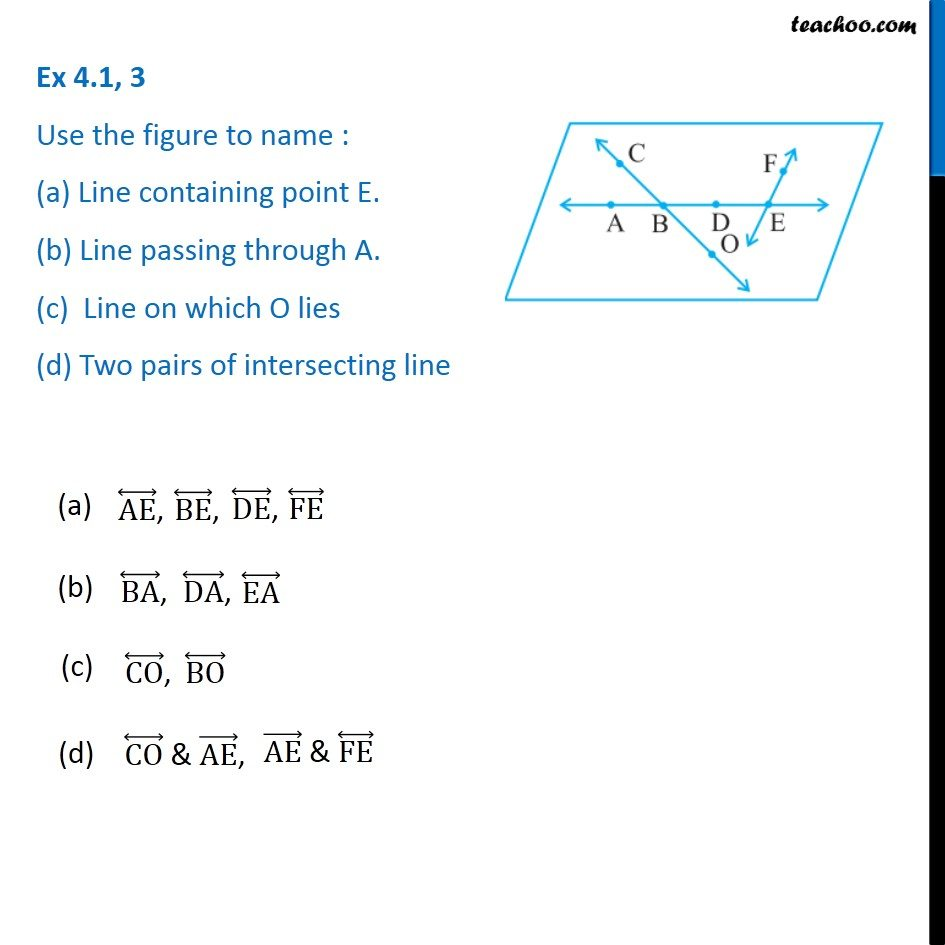1. Chapter 4 Class 6 Basic Geometrical Ideas
2. Concept wise
3. Point, Line, ray, angle

Transcript

Ex 4.1, 3 (Chapter 4 Class 6 NCERT) Use the figure to name: (a) Line containing point E. (b) Line passing through A. (c) Line on which O lies (d) Two pairs of intersecting line The answers are (a) AE, BE, DE, FE (b) BA, DA, EA (c) CO, BO (d) CO & AE, AE & FE

Point, Line, ray, angle